Select Page

Cost of Goods Sold

Cost of goods sold (CGS) includes the costs attributable to the production of the goods sold by company. This amount includes the materials cost used in creating the goods along with the direct labor costs used to produce the good and also includes Factory Overhead (FOH) which are all indirect costs. CGS appears on the income statement and can be deducted from net revenue to calculate a company’s gross profit.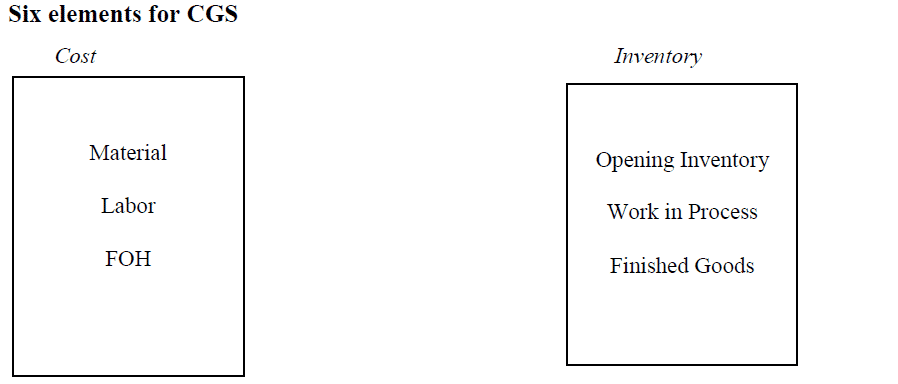Cost of Goods Sold Format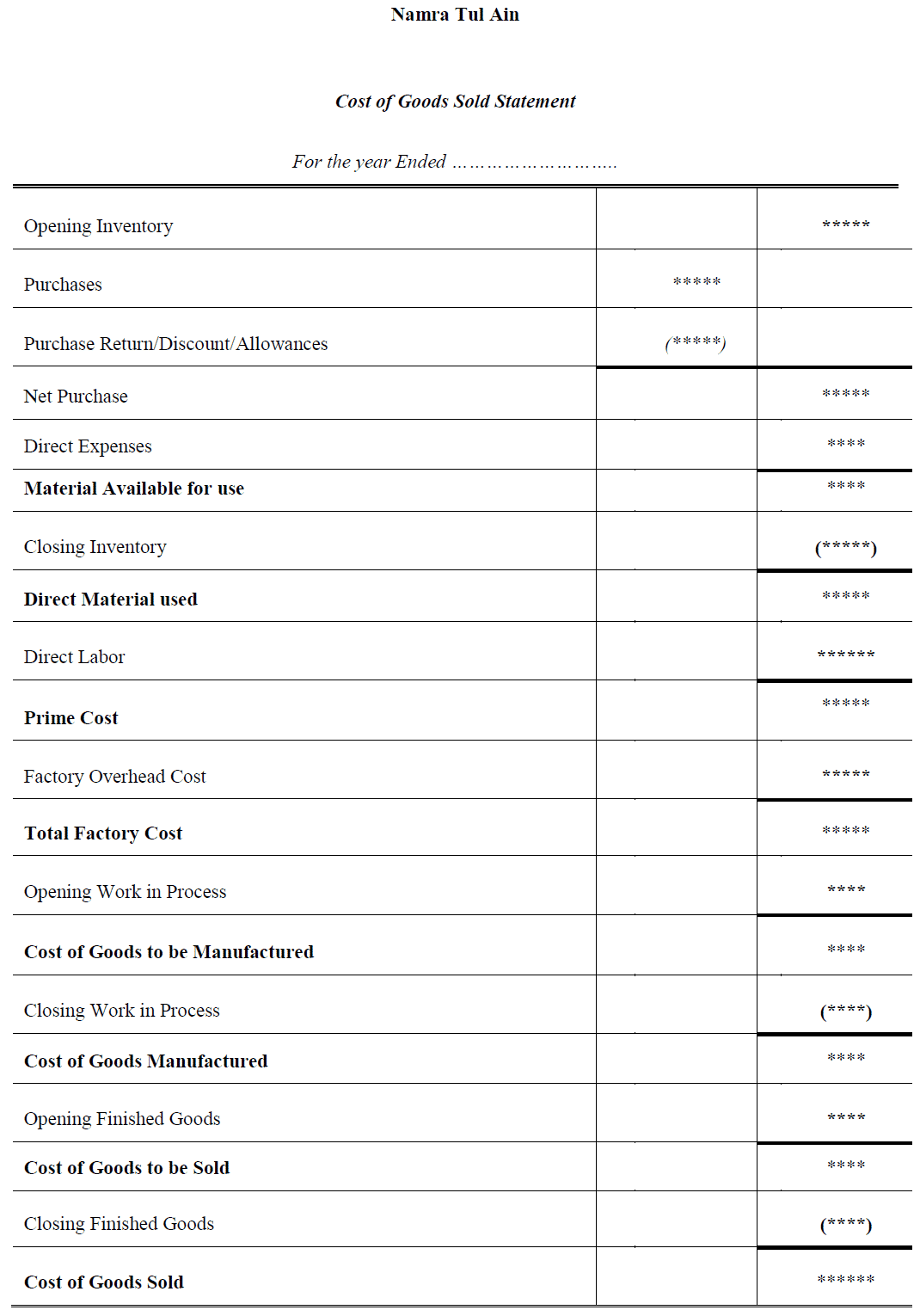>> Practice Cost of Goods Sold Format Download

Important Formulas

1. Direct Material Used = Opening Inventory + Net Purchases (Purchases – all contra purchases) + Direct Expenses – Ending Inventory

2. Prime Cost = Direct Material Cost + Direct Expenses Cost + Direct Labor Cost

3. Total Factory Cost = Direct Material Cost + Direct Expenses + Direct Labor Cost + Factory Overhead Cost (All indirect expense of factory are called FOH)

4. Conversion Cost = Direct Labor Cost + FOH Cost

5.  Cost of Goods Manufactured = Total Factory Cost + Opening Work in Process – Ending Work in Process

6. Cost of Goods Sold = Cost of Goods Manufactured + Opening Finished Goods – Ending Finished Goods

7. Gross Profit/Loss = Net Sales (Sales – all contra sales) – Cost of Goods Sold

8. Operating Profit/Loss= Gross Profit – Commercial Expenses

9. Net Profit/Loss = Operating Profit – Financial Charges – Taxes – Other Expenses + other income

10. Per Unit Cost = Cost of Goods Manufactured / No. of Units Produced

>> Practice Cost of Goods Sold Problems and Solutions.

Example # 1:

The following are the figures relating to the Tooba Mazhar Co. for the month of March, 2010.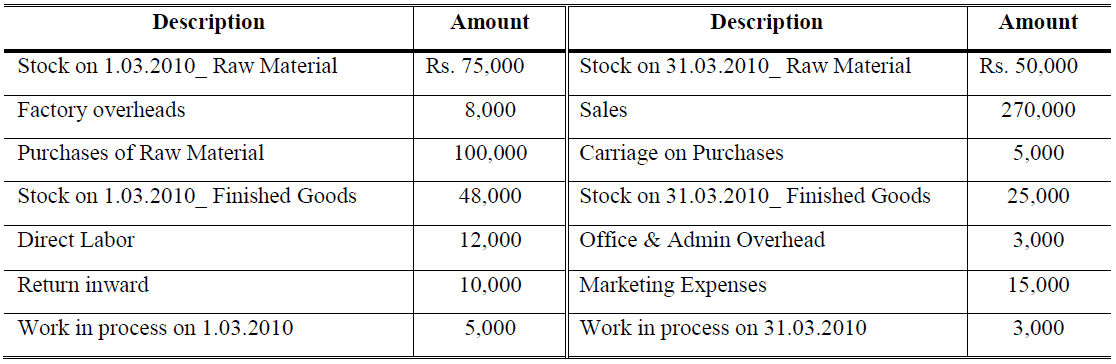Requirement: Prepare statement showing Prime Cost; Conversion Cost; Total Cost; Cost of Goods Manufactured; Cost of Goods Sold. Calculate Gross Profit; Net Profit and per unit cost by assuming that 38,000 units were produced during March.

Solution: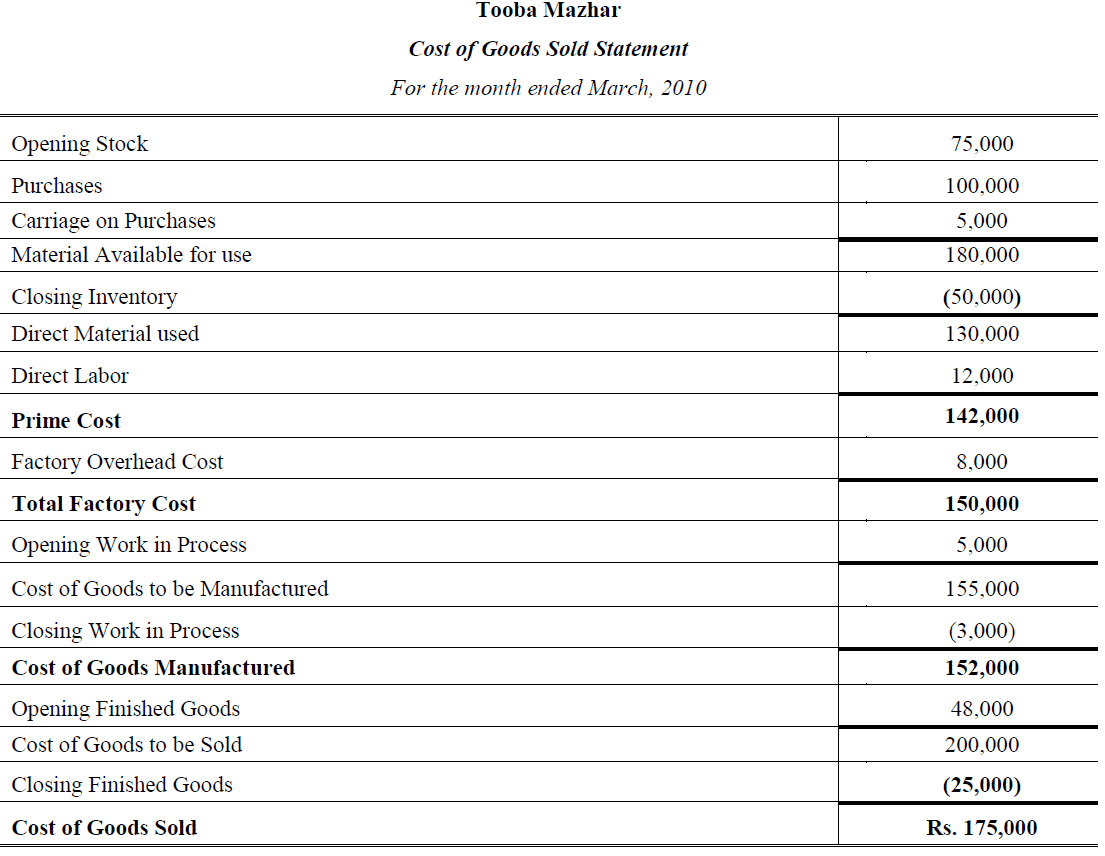Gross Profit = Net Sales – CGS = (270,000 – 10,000) – 175,000 = Rs. 85,000

Net Profit = Gross Profit – indirect expenses of office   =   85,000 – (3,000 + 15,000) = Rs. 67,000

Per Unit Cost = Cost of Goods Manufactured / No. of Units Produced = 152,000 / 38,000 = Rs. 4 Per Unit

Conversion Cost = Direct Labor + FOH                           =                  12,000 + 8,000         = Rs. 20,000

>> Practice Cost of Goods Sold MCQs.

>>> Further Reading Inventory Valuation.

Example # 2:

Following Data related to Aujala Shabbir Manufacturing at the end of April, 2010: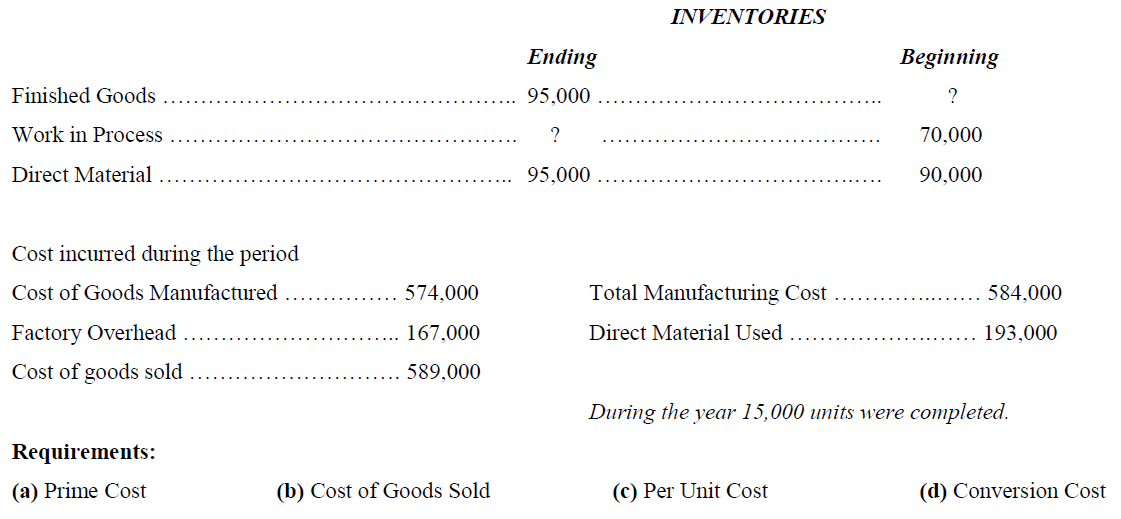Solution: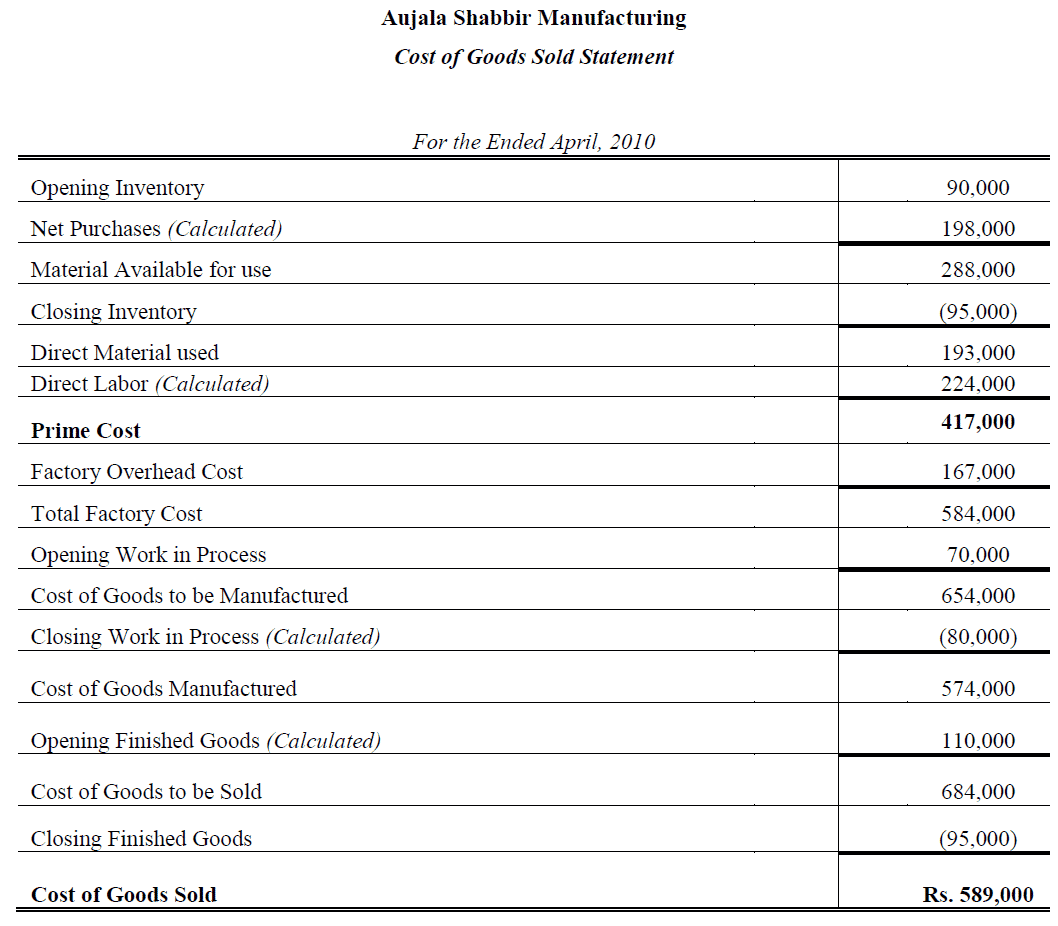Per unit Cost of goods manufactured          =       574,000 / 15,000                     =    Rs. 38 Per Unit

Conversion Cost = Direct Labor + FOH     =          224,000 + 167,000                  =     Rs. 391,000

>> Practice Cost of Goods sold Quiz 1 and Quiz 2.